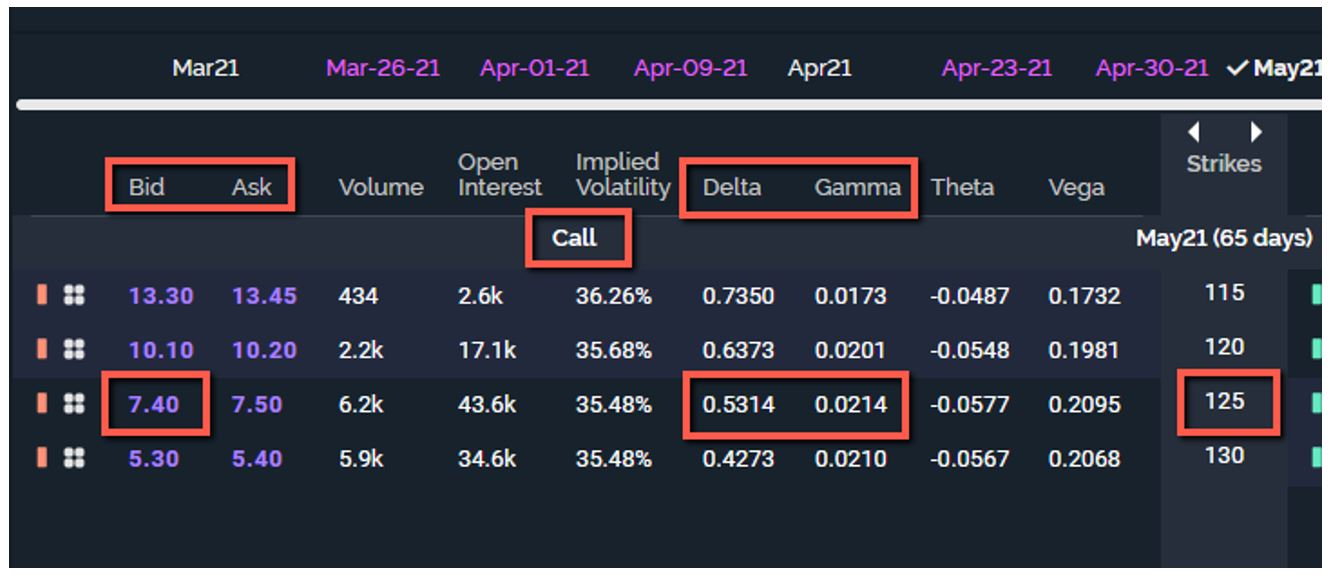# Option Gamma and How It Differs from Option Delta

Although it will always be debatable which is the most important option greek, many option traders point to option delta. This is understandable, considering most new option traders become acquainted with delta first. However, you cannot dismiss option gamma, which is sometimes referred to as a derivative of a derivative.

###### Option Delta and Option Gamma

Option delta measures how much the theoretical value of an option will change if the underlying moves up or down by \$1. Long calls have positive deltas meaning that if the stock gains value so does the option value all constants being equal. Long puts have negative deltas meaning that if the stock gains value the option value will decrease all constants being equal.

Option gamma is the rate of change of an option’s delta relative to a change in the underlying. In other words, option gamma can determine the degree of delta move. Keeping it simple, for a \$1 change in the underlying, delta will change by the amount of gamma. Gamma increases call option deltas when the underlying moves higher and vice versa. Gamma increases put option delta when the underlying moves lower and vice versa.

###### Delta and Gamma Example

Take a look at the option chain below. The position is a short (or sold) 125 call with a delta of approximately 0.53 (rounded down) and a gamma of approximately 0.02 (rounded down as well).In this example, if a call option is priced at 7.40 and has an option delta of 0.53 and the stock moves higher by \$1, the call option should increase in price to 7.93 (7.40 + 0.53). In addition, the new delta of the short call would be 0.55 (0.53 + 0.02) because of the \$1 move higher.

###### Positive and Negative Gamma

When you buy options (calls and puts), you get positive gamma. When you sell options, you get negative gamma. Positive gamma helps your delta both ways. In other words, if you have a position with positive delta and positive gamma and the underlying moves higher, the positive delta will increase and the gains will become incrementally larger. If the underlying moves lower under the same circumstances, the positive delta will decrease so the losses become incrementally smaller based on delta.

Negative gamma, in turn, hurts you both ways. If you have a position with positive delta but negative gamma and the underlying moves higher, the positive delta will decrease and the gains will become incrementally smaller. If the underlying moves lower under the same circumstances, the positive delta will increase so the losses become incrementally larger based on delta.

###### ATM, ITM and OTM Gamma

Option gamma is usually highest for near-term and at-the-money (ATM) strike prices, and it usually declines if the strike price moves more in-the-money (ITM) or out-of-the-money (OTM). As the stock moves up or down, option gamma drops in value because option delta may be either approaching 1.00 or zero. Because option gamma is based on how option delta moves, it decreases as option delta approaches its limits of either 1.00 or zero.

###### Finally

Gamma tends to be one of those greeks (along with vega) that confuses option traders the most. If you remember these few facts about gamma, it can really improve your understanding of all the greeks. I like to tell option traders that delta is the rate of speed for the position and gamma is how quickly it gets there.

John Kmiecik
Senior Options Instructor
Market Taker Mentoring, Inc.